• b = 2; c = 3.5; x1 = fzero(@(x) cubicpoly(x,b,c),0) x2 = fzero(@cubicpoly,0,[],b,c) function y = cubicpoly(x,b,c) ...匿名矩阵的传递参数的思路是再次定义一个函数，将参数传入。 第一种方法比较传
b = 2;
c = 3.5;
x1 = fzero(@(x) cubicpoly(x,b,c),0)
x2 = fzero(@cubicpoly,0,[],b,c)
function y = cubicpoly(x,b,c)
y = x^3 + b*x + c;
end

两种方法的输出内容一致。
x1 =

-1.094475926784920e+00

x2 =

-1.094475926784920e+00

匿名矩阵的传递参数的思路是再次定义一个函数，将参数传入。
第一种方法比较传统。
第二种方法比较简洁。其中空矩阵的作用应该就是分隔符号，前面是主函数，后面是传递参数。


展开全文• matlab支持多输入多输出函数的返回值可以为一个向量，矩阵，或者多个元素。为了让函数可以调用，需要对函数进行命名。 function [输出变量] = 函数名称(输入变量） 即包含： 输入参数 输出参数 函数名 注释 ...
matlab支持多输入多输出，函数的返回值可以为一个向量，矩阵，或者多个元素。为了让函数可以调用，需要对函数进行命名。
function [输出变量] = 函数名称(输入变量）
即包含：
输入参数
输出参数
函数名
注释
函数体
如图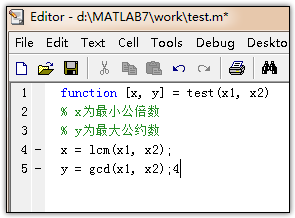、
回到主窗口，在Command Window中，输入test(3,4)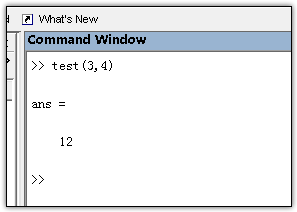也就是输入函数名称和自变量，就会得到因变量，因变量在函数体中，应该和自变量有个关系式，
因变量=一个自变量的式子
而如果我们输入[x, y] = test(3, 4),则显示如下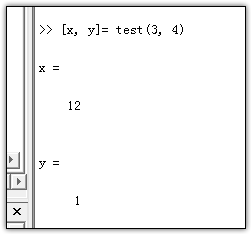这说明如果对于多参数输出的函数，如果没有指明接受参数，那么系统只默认接收第一个参数并存至ans变量中。
展开全文• 下面就总结一下如何在MATLAB定义函数。同时matlab支持多输入多输出，函数的返回值可以为一个向量，矩阵，或者多个元素。为了让函数可以调用，需要对函数进行命名。matlab支持多输入多输出，函...
MATLAB是本人经常使用的程序，编程过程中经常会有部分代码重复出现，为了简化代码许多时候希望将实现特定功能的特定的代码(算法)书写成函数的形式，提高代码的可封装性与重复性，简化代码设计，提高执行效率！下面就总结一下如何在MATLAB中定义函数。同时matlab支持多输入多输出，函数的返回值可以为一个向量，矩阵，或者多个元素。为了让函数可以调用，需要对函数进行命名。matlab支持多输入多输出，函数的返回值可以为一个向量，矩阵，或者多个元素。为了让函数可以调用，需要对函数进行命名。function [输出变量] = 函数名称(输入变量)即包含：输入参数输出参数函数名注释函数体1.首先建立M文件或直接点击(File/New/Function)建立函数文件，其中函数文件的格式是：function [输出变量] = 函数名称(输入变量)% 注释% 函数体2。如下所示，是编写的一个求1到n之和的求和函数 eg_sum，按照上述格式，编写代码如下并保存文件，注意文件命名时不能以数字开头：function [s]  = eg_sum( n )% calculate the sum of 1..ns = 0;for i = 1:ns = s + i;end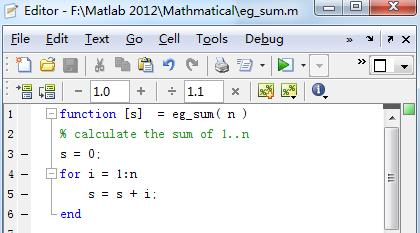3.回到控制台Command Window(主界面),注意要使用之前保存的函数文件名来调用函数，如下所示：>> clear>> a = eg_sum(10)运行结果： a = 55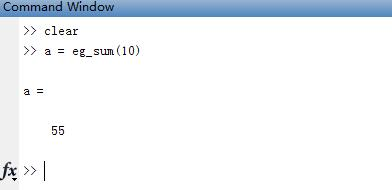4.许多网友说函数名一定要与函数文件名一致，其实不是这样子的，只要知道调用函数时是通过函数文件名调用的这点即可！比如下图所示，即使文件名仍然是eg_sum，但我可以修改函数名为：my_sum 调用时用文件名调用就好，如下所示：>> b = eg_sum(10)运行结果： b = 55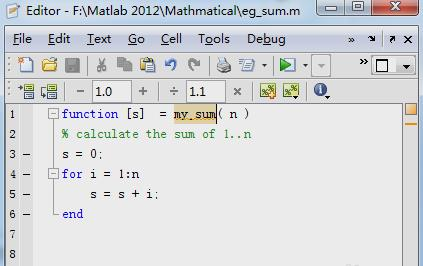5.注意事项：在调用函数时，请确保已将matlab当前目录切换到函数文件所在处，不然会报错：Undefined function 'eg_sum' for input arguments of type 'double'.转自百度经验：huzhijian421
展开全文• 函数文件的基本结构 function 输出形参表=函数名（输入形参表） 注释说明部分 函数体语句 注意：当多个形参时，形参...当输出形参多于一个时，应该用方括号括起来，构成一个输出矩阵函数文件名与函数名自己定义 ...
函数文件的基本结构
function 输出形参表=函数名（输入形参表）
注释说明部分
函数体语句
注意：当多个形参时，形参之间用逗号分隔，组成形参表。当输出形参多于一个时，应该用方括号括起来，构成一个输出矩阵。
函数文件名与函数名自己定义
return语句表示结束函数的执行
调用格式：
[输出实参表]=函数名（输入实参表）
注意函数输入参数称为实际参数，简称实参
例题  写一个求圆面积的公式
function s=s(r)
s=pi*r*r;
disp(s)

>> s(2)
12.5664

ans =

12.5664

匿名函数
格式：
函数变量=@（匿名函数输入参数）匿名函数表达式
例如：
>> f=@(x,y,z)x*y+z

f =

包含以下值的 function_handle:

@(x,y,z)x*y+z

>> f(2,3,4)

ans =

10

函数的嵌套调用
在一个函数中，调用其它函数
函数的递归调用
一个函数调用它自身递归调用
（1）直接递归调用
f函数中调用f函数
（2）间接递归调用
f1函数中调用了f2函数，f2函数中调用了f1函数
例子1  利用函数的递归调用，求n！
思路：
n！=n*（n-1）！直到*1！，1！=1
function f=jiecheng(n)
if n<=1
f=1;
else
f=jiecheng(n-1)*n;
end

>> i=jiecheng(3)

i =

6

此中函数先计算jiecheng（3）输出jiecheng（3）然后又输出jiecheng（2）*3  然后输出jiecheng（1）23最后输出6


展开全文• matlab函数大全-matlab函数大全.doc 比较白痴的东西，不过对初学者也许有用，特分享一下。不要喷我哦!函数具体用法可以用help查一下。附件内容与下面一样的。 Aabs 绝对值、模、字符的ASCII码值 acos 反余弦 ...
• 当输出形参多于一个时，应该用方括号括起来，构成一个输出矩阵函数文件名通常由函数名再加上扩展名.m组成，函数文件名与函数名也可以不相同。 return语句表示结束函数的执行。 调用格式： [输出实参表]=函数名...
• 06-用函数文件的定义与调用 % 第三节 程序流程控制 % 06-用函数文件的定义与调用 % 函数文件的基本结构 % function输出形参表=函数名(输入形参表) % 注释说明部分 % 函数体语句 % 当有多个形参时，形参之间用逗号...object
• 1，矩阵定义和随机生成（magic(A)）,求和（行sum)和转置（A') 2，求矩阵的主对角线之和sum(diag(A)),行列式的值det(A),矩阵的逆(inv(A)),输出分数设置(format rat） 3，求矩阵的逆，求方程的根，矩阵的...
• //本程序定义了一个矩阵相乘的成员函数，该函数需要输入三个参数，分别是要作乘积的两个矩阵和需要一个接收乘积的矩阵 //该成员函数能够对这三个矩阵的维数进行判断，若不符合矩阵的乘法则返回错误信息 //由于本程序...
• 该楼层疑似违规已被系统折叠隐藏此楼查看此楼matlab与vc混合编程中，定义2个矩阵输入输出。假使文件定义为r.c，用,mex -r.c编译之后，假使输入矩阵为a,b,可是执行s=r(a,b)却只能得到第一个outdata0的输出，如果我要...
• 基本结构为： 1.r＝normrnd(mu,sigma)：生成服从正态分布(mu参数代表均值，sigma参数代表...2.r＝normrnd(mu,sigma,m)：生成服从正态分布(mu参数代表均值，sigma参数代表标准差)的 随机数矩阵矩阵的形式由m定义...
• 一、将某MATLAB函数转换为C/C++文件输出 1.新建一个.m文件（这个文件必须是函数文件，函数名和文件名必须一致），在.m文件中把求逆函数写好，如下 2.在MATLAB软件中找到Coder 3.定义输入类型，完成之后点击Next ...
• 函数文件的基本结构 ...在定义函数时，输入、输出参数没有分配空间，所以称之为形势参数。 当多个形参是，形参之间用逗号分隔，组成形参表。当输出形参多于一个时，应该用方括号括起来，构成一个矩阵。 r...
• 最近在使用matlab做代数计算时，通过定义syms 变量和矩阵运算得到了16个代数方程，分别储存在left和right两个4*4矩阵中。每个left(i)=right(i)即为我想要的一个方程。我想用fprint在命令行窗口输出我计算得到的16个...
• MATLAB基础MATLAB的常识MATLAB构建矩阵定义矩阵使用冒号创建矩阵定义特殊矩阵矩阵的索引矩阵的操作操作矩阵函数 MATLAB的常识 编号 1 clc清除命令行 2 clear：清除工作区 3 matlab索引从1开始 4 ...
• exprnd函数，生成服从指数分布的随机数 ...生成服从参数为MU的指数分布的随机数矩阵矩阵的形式由m定义。m是一个1×2向量，其中的两个元素分别代表返回值R中行与列的维数。 R＝exprnd(MU，m，n)  生成m×n形式
• 1.2 matlab函数实现 通过自定义Vector_norm(a,p)函数实现对向量a的1范数、2范数、p范数和inf范数的计算，具体代码如下： function X=Vector_norm(a,p) %%%输入向量a和所求的向量的范数类型（包括1范数,2范数,p范数,...
• 一个基本的原则要记住：字符串中的单引号，用两个单引号来定义（不是一个双引号）！ 1：比如，我想输出 [1 2]’,也就是其转置[1;2],这时就可以使用命令 eval(['disp([1 2]'')']) 执行了矩阵的转置 1 2 2：对比 ...
• 函数定义行（必须由关键字function开头，紧跟着函数输出变量；函数名与变量一样） ②帮助文本的标题行，简称H1行 ③帮助文本的内容，详细说明变量的类型，使用时的语法规则，使用举例和相关的函数名作为...
• %% 初始定义 x1=275;y1=71; x2=299;y2=26.5; X = p(1); Y = p(2); sys= [X;Y];%结果输出 toc %% 自定义函数 function p = CrossPoint(x1,y1,x2,y2,a,b) r = sqrt((x2-x1)^2...
• ## matlab 中括号

千次阅读 2018-01-05 17:23:44
中括号用来构建向量(Vectors)或者是矩阵(Matrices)。如[6.9 9.64 sqrt(-1)] 就是一个有三个元素的向量。[11 12 13; 21 22 23] 是个2*3的矩阵....)用来结束一行。 中括号的另一个作用是在函数中，...2.定义函数的返回
• 2.2 MATLAB矩阵和多维矩阵介绍 12 2.3 MATLAB中的变量 16 2.4 算术表达式和数学函数 19 2.5 计算浮点运算次数和时间管理 25 2.6 输出格式 27 2.7 帮助命令和示范 28 2.8 保存和装载 31 2.9 命令文件和函数文件 32 ...
• 1，函数名称定义要求必须以字符开头，后面可以用字符，下划线和数字组成函数的名称。 2，MATLAB函数名称的长度有限制，用户可以用namelengthmax函数获取相应的值，如果超过了这个值，MATLAB取此之前的作为函数名称...
• 1. mesh(Z)语句mesh(Z)语句可以给出矩阵Z元素的三维消隐图，网络表面由Z坐标点定义，与前面叙述的x-y平面的线格相同，图形由邻近的点连接而成．...最后用mesh函数输出．下面我们绘制sin(r)/r函数的图形...
• 本文是仿照这篇文章而来的：《VC调用matlab定义的.m文件中的函数的实例》他的文章中使用的是VC6.0 + MATLAB6.5，有的东西已经不能使用了，因此我这里再写一篇如何用VC2005来调用MATLAB2009a中编写的m文件。...
• CAE仿真工作之中，完成的有限元模型需要定义边界条件，定义载荷激励，定义工况，定义输出卡片，定义求解器等，非常繁琐，对于一些简单的模态求解的卡片定义还好说，但如果对于大量的传递函数卡片定义来说，就非常的...
• 该楼层疑似违规已被系统折叠隐藏此楼查看此楼matlab与vc混合编程中，定义2个矩阵输入输出。假使文件定义为r.c，用,mex -r.c编译之后，假使输入矩阵为a,b,可是执行s=r(a,b)却只能得到第一个outdata0的输出，如果我要...
• 1. mesh(Z)语句 mesh(Z)语句可以给出矩阵Z元素的三维消隐图，网络表面由Z坐标点定义，与前面叙述的x-y平面的线格相同，图形由邻近的点连接而成．...最后用mesh函数输出． 下面我们绘制sin(r)/...# matlab定义函数输出矩阵matlab 订阅